Open In App
Related Articles
• NCERT Solutions for Class 9 Maths

# Class 9 NCERT Solutions- Chapter 8 Quadrilaterals – Exercise 8.1

### Question 1. The angles of quadrilateral are in the ratio 3:5:9:13. Find all the angles of the quadrilateral.

Solution:

As we know that, the sum of the angles of a quadrilateral is 360° (Angle sum property of quadrilateral)

As they are in ratio 3 : 5 : 9 : 13, so we can assume angles be as 3x, 5x, 9x and 13x.

So,

3x + 5x + 9x + 13x = 360°

30x = 360°

x = 360/30 = 12°

So the angles will be as follows:

3x = 3×12 = 36°

5x = 5×12 = 60°

9x = 9×12 = 108°

13x = 13×12 = 156°

Hence, the angles of quadrilateral are 36°, 60°, 108° and 156°.

### Question 2. If the diagonals of a parallelogram are equal, then show that it is a rectangle.

Solution:

As mentioned parallelogram, so let PQRS be a parallelogram

where, given

PR = QS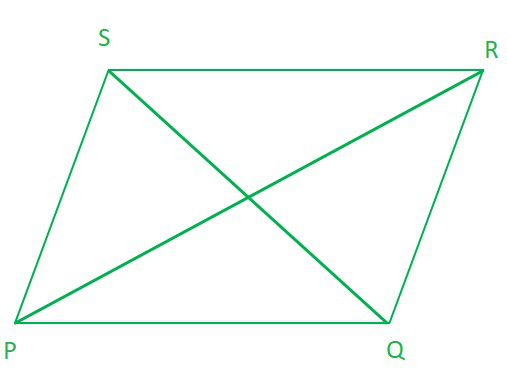In ∆PQR and ∆QRS,

PR = QS      …………………[Given]

PQ = RS      …………………[Opposite sides of a parallelogram]

QR = RQ     …………………[Common side]

∴ ∆PQR ≅ ∆QRS           [By SSS congruency]

so, ∠PQR = ∠QRS [By C.P.C.T.] ………………………………………….(1)

Now, PQ || RS and QR is a transversal. …………………….[PQRS is a parallelogram]

∴ ∠PQR + ∠QRS = 180°  [Co-interior angles of parallelogram]…………………………………… (2)

From (1) and (2), we have

∠PQR = ∠QRS = 90°

i.e., PQRS is a parallelogram having an angle equal to 90°.

Hence, PQRS is a rectangle. (having all angles equal to 90° and opposite sides are equal)

### Question 3. Show that if the diagonals of a quadrilateral bisect each other at right angles, then it is a rhombus.

Solution:

where, given

PO = RO

SO = QO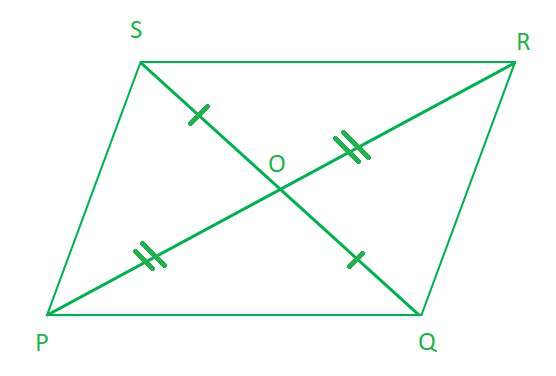In ∆POQ and ∆POS, we have

PO = PO [Common]

OQ = OS [O is the mid-point of QS]

∠POQ= ∠POS [Each 90°]

∴ ∆POQ ≅ ∆POS [By, SAS congruency]

∴ PQ = PS [By C.P.C.T.] ……..    (1)

Similarly, PQ = QR …………………..(2)

QR = RS ……………………………………..(3)

RS = SP ………………………………………(4)

∴ From (1), (2), (3) and (4), we have

PQ = QR = RS = SP

Thus, the quadrilateral PQRS is a rhombus.

Alternative Solution:

So as it is given that diagonals of a quadrilateral PQRS bisect each other

According to Theorem 8.7 NCERT it is a parallelogram

PQRS can be proved first a parallelogram then proving one pair of adjacent sides equal will result in rhombus.

In ∆POQ and ∆POS, we have

PO = PO [Common]

OQ = OS [O is the mid-point of QS]

∠POQ= ∠POS [Each 90°]

∴ ∆POQ ≅ ∆POS [By,SAS congruency]

∴ PQ = PS [By C.P.C.T.] ……..    (1)

Similarly, PQ = QR …………………..(2)

QR = RS ……………………………………..(3)

RS = SP ………………………………………(4)

From (1), (2), (3) and (4), we have

PQ = QR = RS = SP

Hence, as a parallelogram has all sides equal then it is called a rhombus.

### Question 4. Show that the diagonals of a square are equal and bisect each other at right angles.

Solution:

As it is mentioned that it is a square so,

all sides are equal. (PQ = QR = RS = SP)

all angles at four corners = 90°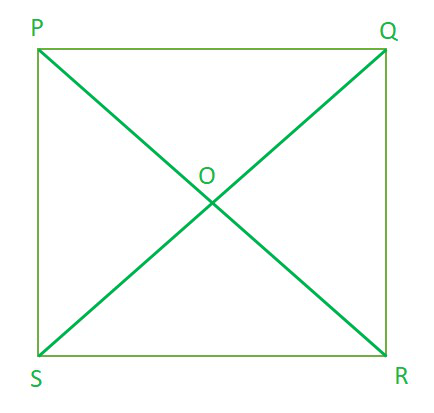So, to prove that the diagonals are equal, we need to prove PR = QS.

In ∆PQR and ∆QPS, we have

PQ = QP ……………….[Common]

PS = PQ ………………..[Sides of a square PQRS]

∠PQR = ∠QPS …….[Each angle is 90°]

∆PQR ≅ ∆QPS [By SAS congruency]

AC = BD [By C.P.C.T.] …(1)

Now as we know square is also a parallelogram

In ∆POQ and ∆ROS, we have

∠POQ = ∠ROS ……………….[Opposite angles of two intersecting lines]

PQ = RS ………………..[Sides of a square PQRS]

∠PQO = ∠RSO …….[Alternate interior angles are equal]

∆PQR ≅ ∆QPS [By ASA congruency]

OP = OQ = OR = OS (Hence the diagonals are equal and bisect each other)

Now , In ∆OQP and ∆OSP, we have

OQ = OS [Proved]

QP = SP [Sides of a square PQRS]

OP = OP [Common]

∆OQP ≅ ∆OSP [By SSS congruency]

∠POQ = ∠POS [By C.P.C.T.] …(3)

∠POQ + ∠POS = 180° (∵ ∠POQ and ∠POS form a linear pair)

∠POQ = ∠POS = 90° [By(3)]

PR ⊥ QS …(4)

From (1), (2) and (4), we get PR and QS are equal and bisect each other at right angles (90°).

### Question 5. Show that if the diagonals of a quadrilateral are equal and bisect each other at right angles, then it is a square.

Solution:

where,

PR = QS

OP = OR = OQ = OS

∠POQ = ∠QOR = ∠ROS = ∠SOP = 90°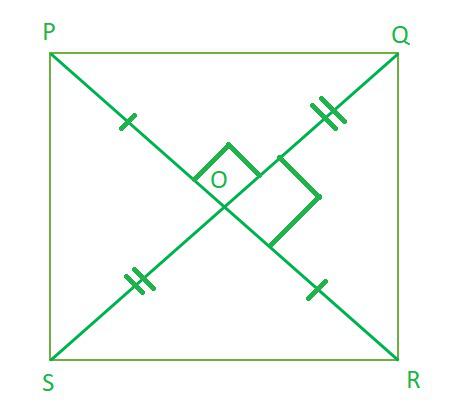Now, in ∆POS and ∆POQ, We have

∠POS = ∠POQ [Each 90°]

PO = PO [Common]

OS= OQ [ ∵ O is the midpoint of BD]

∆POS ≅ ∆POQ [By SAS congruency]

PS = PQ [By C.P.C.T.] …(1)

Similarly, we have

PQ = QR … (2)

QR = RS …(3)

RS = SP…(4)

From (1), (2), (3) and (4), we have

PQ = QR = RS = SP

Hence, Quadrilateral PQRS have all sides equal.

Now, In ∆POS and ∆ROQ, we have

PO = RO [Given]

OS = OQ [Given]

∠POS = ∠ROQ [Vertically opposite angles]

So, ∆POS ≅ ∆ROQ [By SAS congruency]

∠PSO = ∠RQO [By C.P.C.T.]

So as they form a pair of alternate interior angles.

PS || QR

Similarly, PQ || RS

PQRS is a parallelogram.

Parallelogram having all its sides equal is a rhombus.

PQRS is a rhombus.

Now, in ∆PQR and ∆QPS, we have

PR = QS [Given]

QR = SP [Proved]

PQ = QP [Common]

∆PQR ≅ ∆QPS [By SSS congruency]

∠PQR = ∠QPS [By C.P.C.T.] ……(5)

Since, PS || QR and PQ is a transversal.

∠PQR + ∠QPS = 180° .. .(6) [ Co – interior angles]

∠PQR = ∠QPS = 90° [By(5) & (6)]

So, rhombus PQRS is having corners angle equal to 90°.

Hence, PQRS is a square.

### Question 6. Diagonal AC of a parallelogram ABCD bisects ∠A (see Fig. 8.19). Show that

(i) it bisects ∠C also,

(ii) ABCD is a rhombus.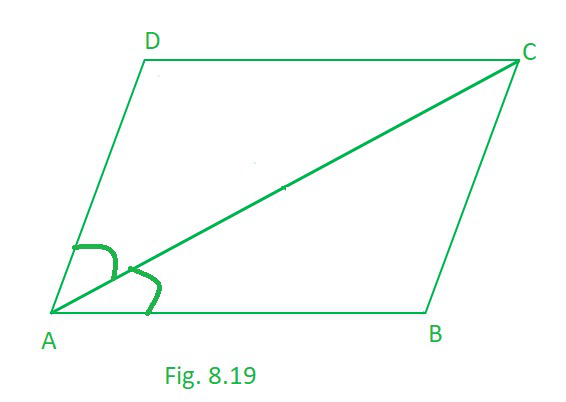Solution:

(i) As, ABCD is a parallelogram.

∠BAC = ∠DCA ……………………………(1) [Alternate interior angles are equal]

∠CAD = ∠BCA ………………………….(2) [Alternate interior angles are equal]

Also, ∠CAD = ∠CAB …………………….(3) [ (Given) as AC bisects ∠A]

From (1), (2) and (3), we have

∠DCA = ∠BCA

Hence, AC bisects ∠C.

(ii) In ∆ABC,

∠BAC = ∠DCA ………………………….. [Alternate interior angles are equal]

BC = AB …………………………….(4) [ Sides opposite to equal angles of a ∆ are equal]

But, ABCD is a parallelogram. [Given]

AB = DC ………………….(6) (opposite sides of parallelogram)

From (4), (5) and (6), we have

AB = BC = CD = DA

As, ABCD is a parallelogram having all sides equal then it is a rhombus.

### Question 7. ABCD is a rhombus. Show that diagonal AC bisects ∠ A as well as ∠ C and diagonal BD bisects ∠ B as well as ∠ D.

Solution:

As, ABCD is a rhombus, so

AB = BC = CD = DA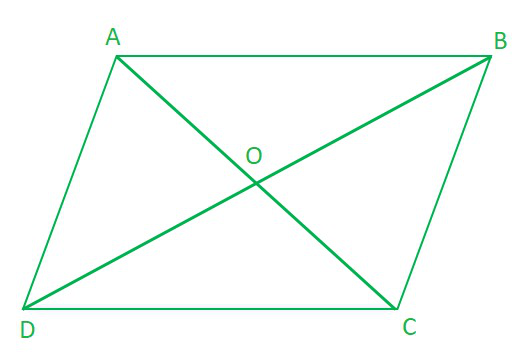∠ADB= ∠ABD……………………….(1) [Angles opposite to equal sides of a triangle are equal]

∠ADB = ∠CBD …………………..(2) [ ∵ Alternate interior angles are equal] (Rhombus is a parallelogram)

From (1) and (2), we have

∠CBD = ∠ABD …………………..(3)

∠ABD= ∠CDB ……………………..(4)      [ ∵ Alternate interior angles are equal]

From (1) and (4),

Hence, BD bisects ∠B as well as ∠D.

Similarly, we can prove that AC bisects ∠C as well as ∠A..

### Question 8. ABCD is a rectangle in which diagonal AC bisects ∠ A as well as ∠ C. Show that:

(i) ABCD is a square

(ii) diagonal BD bisects ∠ B as well as ∠ D.

Solution:

There is rectangle ABCD such that AC bisects ∠A as well as ∠C, so

∠BAC = ∠DAC and,

∠DCA = ∠BCA ………………………………(1)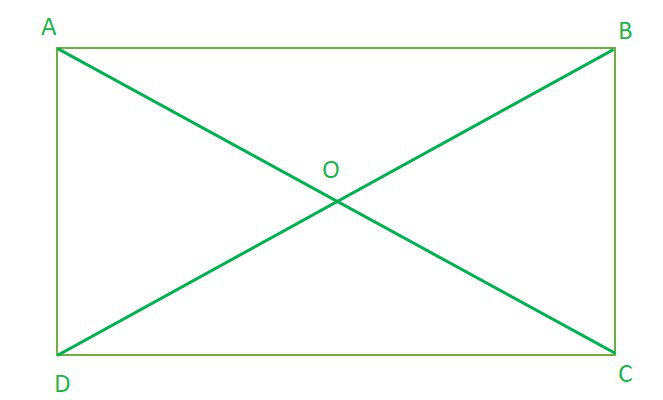(i) As we know that every rectangle is a parallelogram.

ABCD is a parallelogram.

∠BCA = ∠DAC …………………….(2) [ Alternate interior angles are equal]

From (1) and (2), we have

∠DCA= ∠DAC……………………….(3)

In ∆ABC, ∠DCA= ∠DAC then,

CD = DA [Sides opposite to equal angles of a ∆ are equal]

Similarly, AB = BC

So, ABCD is a rectangle having adjacent sides equal.

ABCD is a square.

(ii) Since, ABCD is a square

AB = BC = CD = DA

so, In ∆ABD, as AB = AD

∠ABD = ∠ADB [Angles opposite to equal sides of a ∆ are equal]……………………..(1)

Similarly, ∠CBD = ∠CDB…………………..(2)

∠CBD = ∠ADB               [Alternate interior angles are equal]………………(3)

From (1) and (3)

∠CBD = ∠ABD

From (2) and (3)

So, BD bisects ∠B as well as ∠D.

### Question 9. In parallelogram ABCD, two points P and Q are taken on diagonal BD such that DP = BQ (see Fig. 8.20). Show that :

(i) ∆APD ≅ ∆CQB

(ii) AP = CQ

(iii) ∆AQB ≅ ∆CPD

(iv) AQ = CP

(v) APCQ is a parallelogram

Solution:

ABCD is a parallelogram

DP = BQ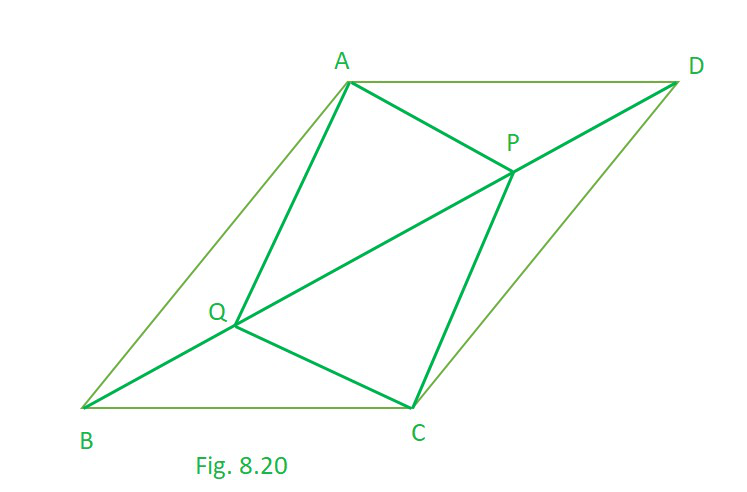(i) As ABCD is a parallelogram

∠ADB = ∠CBD [Alternate interior angles are equal]……………….(1)

∠ABD = ∠CDB   [Alternate interior angles are equal]…………………(2)

Now, in ∆APD and ∆CQB, we have

AD = CB [Opposite sides of a parallelogram ABCD are equal]

PD = QB [Given]

Hence, ∆APD ≅ ∆CQB [By SAS congruency]

(ii) As, ∆APD ≅ ∆CQB [Proved]

AP = CQ [By C.P.C.T.]…………………(3)

(iii) Now, in ∆AQB and ∆CPD, we have

QB = PD [Given]

∠ABQ = ∠CDP [Proved]

AB = CD [ Opposite sides of a parallelogram ABCD are equal]

Hence, ∆AQB ∆CPD [By SAS congruency]

(iv) As, ∆AQB ≅ ∆CPD [Proved]

AQ = CP [By C.P.C.T.] …………………………..(4)

Opposite sides are equal. [From (3) and (4)]

Hence, APCQ is a parallelogram. (NCERT Theorem 8.3)

### Question 10. ABCD is a parallelogram and AP and CQ are perpendiculars from vertices A and C on diagonal BD (see Fig. 8.21). Show that

(i) ∆APB ≅ ∆CQD

(ii) AP = CQ

Solution:

ABCD is a parallelogram

DP = BQ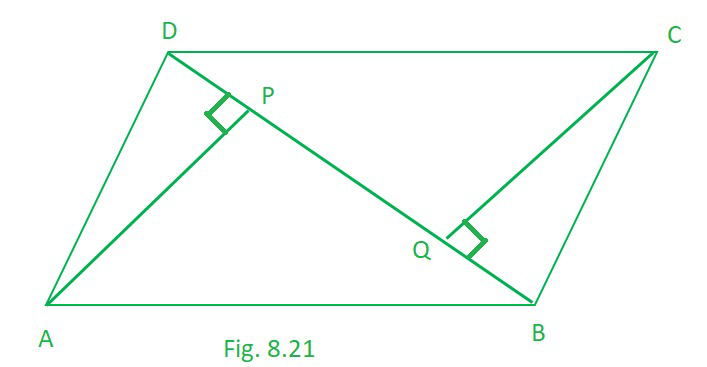(i) In ∆APB and ∆CQD, we have

∠APB = ∠CQD [Each 90°]

AB = CD [ Opposite sides of a parallelogram ABCD are equal]

∠ABP = ∠CDQ [Alternate angles are equal as AB || CD and BD is a transversal]

Hence, ∆APB  ≅  ∆CQD [By AAS congruency]

(ii) As, ∆APB ≅ ∆CQD [Proved]

AP = CQ [By C.P.C.T.]

### Question 11. In ∆ ABC and ∆ DEF, AB = DE, AB || DE, BC = EF and BC || EF. Vertices A, B and C are joined to vertices D, E and F respectively (see Fig. 8.22). Show that

(i) quadrilateral ABED is a parallelogram

(ii) quadrilateral BEFC is a parallelogram

(iv) quadrilateral ACFD is a parallelogram

(v) AC = DF

(vi) ∆ABC ≅ ∆DEF.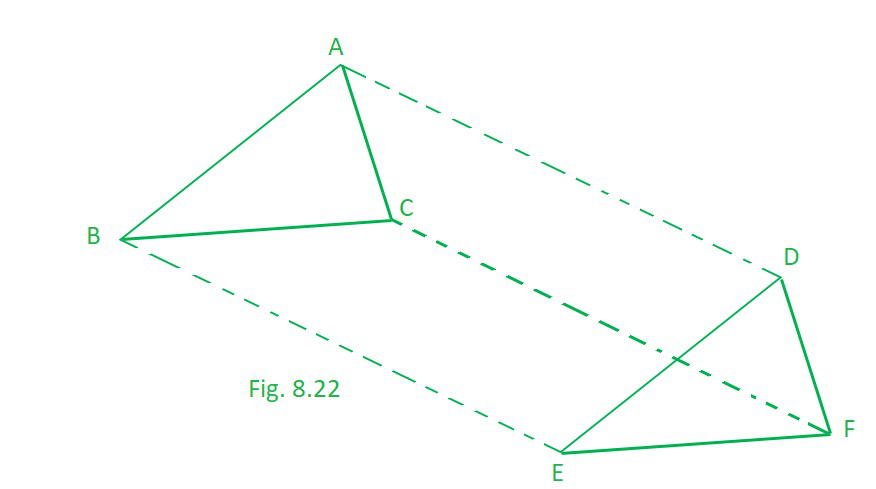Solution:

AB = DE, and AB || DE,

BC = EF and BC || EF

(i) We have AB = DE and AB || DE [Given]

so here., ABED is a quadrilateral in which a pair of opposite sides (AB and DE) are parallel and of equal length.

Hence, ABED is a parallelogram.

(ii) BC = EF and BC || EF [Given]

i.e. BEFC is a quadrilateral in which a pair of opposite sides (BC and EF) are parallel and of equal length.

Hence, BEFC is a parallelogram.

(iii) as, ABED is a parallelogram [Proved]

∴ AD || BE and AD = BE …(1) [Opposite sides of a parallelogram are equal and parallel]

Also, BEFC is a parallelogram. [Proved]

BE || CF and BE = CF …(2) [Opposite sides of a parallelogram are equal and parallel]

From (1) and (2), we have

i.e., In quadrilateral ACFD, one pair of opposite sides (AD and CF) are parallel and of equal length.

Hence, Quadrilateral ACFD is a parallelogram.

(v) Since, ACFD is a parallelogram. [Proved]

So, AC =DF [Opposite sides of a parallelogram are equal]

(vi) In ∆ABC and ∆DEF, we have

AB = DE [Given]

BC = EF [Given]

AC = DE [Proved in (v) part]

∆ABC ≅ ∆DEF [By SSS congruency]

### Question 12. ABCD is a trapezium in which AB || CD and AD = BC (see Fig. 8.23). Show that

(i) ∠A = ∠B

(ii) ∠C = ∠D

(iv) diagonal AC = diagonal BD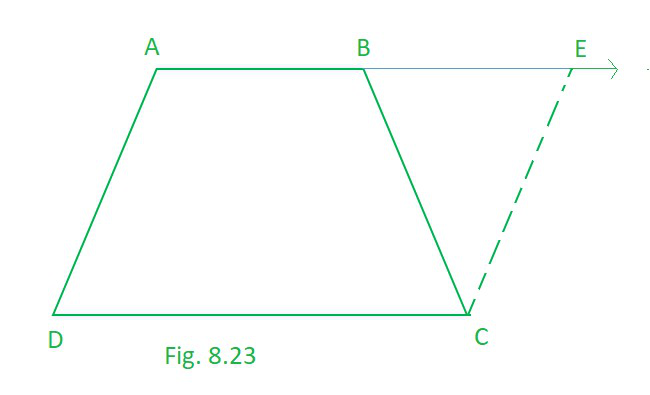[Hint: Extend AB and draw a line through C parallel to DA intersecting AB produced at E.]

Solution:

so basically here is a trapezium ABCD in which AB || CD and AD = BC.

Extended AB and draw a line through C parallel to DA intersecting AB produced at E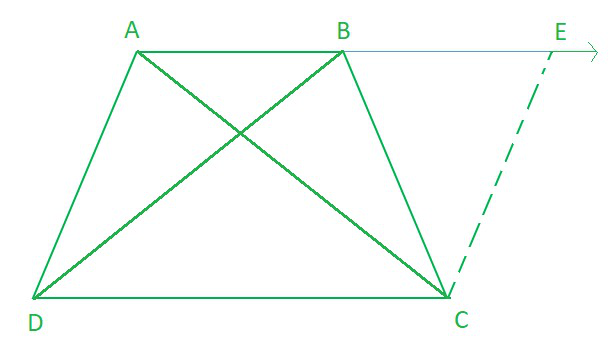(i) AB || DC , AE || DC Also AD || CE

then, AECD is a parallelogram.

AD = CE …………………………(1) [Opposite sides of the parallelogram are equal]

But AD = BC …(2) [Given]

From (1) and (2),

BC = CE

Now, in ∆BCF, we have BC = CF

∠CEB = ∠CBE …(3)  [Angles opposite to equal sides of a triangle are equal]

Also, ∠ABC + ∠CBE = 180° … (4) [Linear pair]

and ∠A + ∠CEB = 180° …(5) [Co-interior angles of a parallelogram ADCE]

From (4) and (5), we get

∠ABC + ∠CBE = ∠A + ∠CEB

∠ABC = ∠A [From (3)]

∠B = ∠A …(6)

(ii) AB || CD and AD is a transversal.

∠A + ∠D = 180° …(7) [Co-interior angles in parallelogram]

Similarly, ∠B + ∠C = 180° … (8)

From (7) and (8), we get

∠A + ∠D = ∠B + ∠C

∠C = ∠D [From (6)]

(iii) In ∆ABC and ∆BAD, we have

AB = BA [Common]